## Extending R’s functionality with packages

While R has a rich suite of functions to work off of, the R community extends on R’s built-in functions by offering customized functions via packages. There are more than 14,000 packages with additional packages being added weekly or daily. Most packages can be downloaded via CRAN (the Comprehensive R Archive Network). Packages are installed in your local user profile. You can install packages by command line via install.packages. In today’s workshop, we’ll make use of five packages: readxl, dplyr, stringr, writexl and ggplot2.

install.packages(c("readxl", "dplyr", "stringr", "writexl", "ggplot2" ))

Installing packages onto your computer does not mean that their functions are instantly available for use in an R session. You need to explicitly tell R which packages are to be used to perform a specified set of tasks.

In this next exercise, we will load the readxl package into our current R session using the library() function.

library(readxl)

The readxl packages contain a function, read_excel, that will allow us to load an Excel file. In this next code chunk, we’ll read the same spring course list loaded yesterday but this time we’ll load it as an excel file.

dat <- read_excel("SP1819.xlsx", sheet = "sheet1")
str(dat)
Classes 'tbl_df', 'tbl' and 'data.frame':   717 obs. of  13 variables:
$Course : chr "AA118" "AA223" "AA223" "AA231" ...$ Section  : chr  "A" "A" "A" "A" ...
$Cr : chr "2" "4" "4" "4" ...$ Days     : chr  "TR" "TR" "TR" "MW" ...
$Times : chr "2:30pm- 4:00pm" "8:00am- 9:30am" "9:45am-10:45am" "11:00am-12:15pm" ...$ Title    : chr  "Dance Technique Lab: Dance Forms of the African Diaspora: Hip-hop (See TD118)" "Critical Race Feminisms and Tap Dance (See WG223)" "Critical Race Feminisms and Tap Dance (See WG223)" "Caribbean Cultures (See AY231)" ...
$DistReq : chr NA "A" "A" NA ...$ Diversity: chr  NA "U" "U" "I" ...
$Room : chr NA NA NA NA ...$ Reg      : num  0 0 0 0 0 0 0 0 0 0 ...
$Max : num NA NA NA NA NA NA NA NA NA NA ...$ Exam     : num  NA NA NA NA NA NA NA NA NA NA ...
\$ Faculty  : chr  "Akuchu" "Thomas, S" "Thomas, S" "Bhimull" ...

## Manipulating data tables

While base R is certainly capable of satisfying most data table manipulation needs, the dplyr and stringr packages (part of a set of packages called the tidyverse) provide a consistent and user friendly platform for working with data tables.

### Subsetting data table by row

First, we’ll load the dplyr package.

library(dplyr)

Next, we’ll subset the spring courses schedule by keeping just those rows whose Days column equals M. Note that R is case sensitive so be sure to specify uppercase M and not lower case m.

dat1 <- dat %>%
filter(Days == "M")

It’s best to picture the data manipulation workflow as an assembly line where the data table is being piped from one station to another where each station is performing an operation on the data table. Here, the piping operator is assigned the %>% glyph–its shortcut key is ctrl+shift+M (or command+shift+M on a Mac). The filter() function is a station along the assembly line that subsets the table by row based on the condition that the value in the Days column equals M. The output of this operation is then passed to a new data table object called dat1.

We can apply multiple conditions to the filter function by piecing the conditions with boolean operators. For example, to filter the table by rows where Days equals M and where Reg is greater than 16, type:

dat1 <- dat %>%
filter(Days == "M" & Reg > 16)

Here, since both conditions must be met, we tie these conditions together with the boolean AND, &. You can also write the above as:

dat1 <- dat %>%
filter(Days == "M",  Reg > 16)

Here, the comma , implies that each condition must be met.

Another boolean operator you might find yourself using is the OR , |, operator. For example, if you want to filter by rows where Days equals M OR MW, type:

dat1 <- dat %>%
filter(Days == "M" | Days == "MW")

When combined with the Reg condition, we get:

dat1 <- dat %>%
filter(Days == "M" | Days == "MW",
Reg > 16)

It’s good practice to split the different conditions across multiple lines to facilitate readability.

### Subsetting data table by column

Using the same piping operation framework, we can subset our table by column names. For example, to limit the table to the columns Course, Days, Times and Reg, type:

dat1 <- dat %>%
select(Course, Days, Times, Reg)

We can, of course, combine data table operations. For example, to combine the row selection operation with this column selection operation, type:

dat1 <- dat %>%
select(Course, Days, Times, Reg) %>%
filter(Days == "M" | Days == "MW",
Reg > 16)

Be careful with the order in which the operations are written out. For example, had we chosen not to keep the Days column in the final output, the following would have generated an error message.

dat1 <- dat %>%
select(Course, Times, Reg) %>%
filter(Days == "M" | Days == "MW",
Reg > 16)
Error in ~Days == "M" | Days == "MW": object 'Days' not found

Going back to our assembly line metaphor, the filter station only sees what’s being passed to it (here, a subset of the original table with the omitted Days column). So in this example, the order in which the operations takes place matters. The filter operation should be placed before the columns selection operation as in:

dat1 <- dat %>%
filter(Days == "M" | Days == "MW",
Reg > 16) %>%
select(Course, Times, Reg)

### Summarizing table columns

The simplest summary is the count. For example, we can extract the total number of rows in the output table using the count() function:

dat %>%
select(Course, Days, Times, Reg) %>%
filter(Days == "M" | Days == "MW",
Reg > 16) %>%
count()
# A tibble: 1 x 1
n
<int>
1    36

We can also summarize columns using descriptive statistics such as the mean, minimum and maximum. Next, we’ll make use of the summarise() function. For example:

dat %>%
select(Course, Days, Times, Reg) %>%
filter(Days == "M" | Days == "MW",
Reg > 16) %>%
summarise(mean_reg = mean(Reg), max_reg = max(Reg))
# A tibble: 1 x 2
mean_reg max_reg
<dbl>   <dbl>
1     26.3     100

Here, the summarise function generates two columns named mean_reg and max_reg. These are names we’ve chosen. The functions that perform the actual statistical summaries are mean() and max().

Sometimes, the data table may contain missing values (denoted as NA). This can be problematic when computing a statistical summary.

mean(c(34.2, 4, NA, 100))
 NA

If an NA is present in a vector, the mean() function will return NA. To have the mean() function ignore the NAs, simply add the argument na.rm = TRUE to the function.

mean(c(34.2, 4, NA, 100), na.rm = TRUE)
 46.06667

This fix applies to other statistical functions such as min(), max() and median()

### Getting row counts by grouped values

We can instruct R to break down summaries by unique column values. For example, to break down the summary statistics by unique Days values (there are only two: M and MW), add the group_by() function before calling the summarise() function.

dat %>%
select(Course, Days, Times, Reg) %>%
filter(Days == "M" | Days == "MW",
Reg > 16) %>%
group_by(Days) %>%
summarise(mean_reg = mean(Reg), max_reg = max(Reg))
# A tibble: 2 x 3
Days  mean_reg max_reg
<chr>    <dbl>   <dbl>
1 M         19.8      25
2 MW        28.8     100

### Splitting a column into multiple columns

In this next exercise, we will split the course number into its program/department two letter designation and its numeric component. These values will be assigned to two new columns that we’ll name Dept and Number. Here, we’ll make use of the stringr package which has a rich set of character manipulating functions.

library(stringr)
dat1 <- dat %>%
select(Course, Days, Times, Reg) %>%
filter(Days == "M" | Days == "MW",
Reg > 16) %>%
mutate(Dept = str_sub(Course, start = 1, end = 2),
Number = str_sub(Course, start = 3, end = 5),
Number = as.integer(Number))

We make use of the mutate() function to create the two new columns. We also make use of the str_sub() function to extract characters based on their position in the string. The position values are inclusive.

Note that the output of str_sub is always a character data type. This does not make sense for the Number variable. We therefore coerce it into an integer by replacing it with its numeric representation.

### Joining tables

Next, we will load another table: Divisions.csv.

div <- read.csv("Divisions.csv", stringsAsFactors = FALSE)
div
                    Division DeptProg
1                 Humanities       AR
2                 Humanities       CL
3                 Humanities       EA
4                 Humanities       EN
5                 Humanities       FR
6                 Humanities       IT
7                 Humanities       GM
8                 Humanities       RU
9                 Humanities       MU
10                Humanities       PL
11                Humanities       RE
12                Humanities       SP
13                Humanities       TD
14                Humanities       JA
15                Humanities       GK
16                Humanities       LT
17                Humanities       CN
18                Humanities       WP
19           Social Sciences       AY
20           Social Sciences       EC
21           Social Sciences       GO
22           Social Sciences       HI
23           Social Sciences       PS
24           Social Sciences       SO
25          Natural Sciences       BI
26          Natural Sciences       BC
27          Natural Sciences       CH
28          Natural Sciences       CS
29          Natural Sciences       GE
30          Natural Sciences       MA
31          Natural Sciences       SC
32          Natural Sciences       PH
33          Natural Sciences       AS
34 Interdisciplinary Studies       AA
35 Interdisciplinary Studies       AM
36 Interdisciplinary Studies       CI
37 Interdisciplinary Studies       ED
38 Interdisciplinary Studies       ES
39 Interdisciplinary Studies       GS
40 Interdisciplinary Studies       JS
41 Interdisciplinary Studies       LA
42 Interdisciplinary Studies       ST
43 Interdisciplinary Studies       WG

The div table consists of the departments’ two letter designation and their affiliated division. We want to add the division names to each course in the dat1 table. To do this, we’ll join the contents of the div table to the dat1 table by matching column values: the Depth column from the dat1 table will be matched to the DeptProg column from the div table.

dat1 <- dat %>%
select(Course, Days, Times, Reg) %>%
filter(Days == "M" | Days == "MW",
Reg > 16) %>%
mutate(Dept = str_sub(Course, start = 1, end = 2),
Number = str_sub(Course, start = 3, end = 5),
Number = as.integer(Number)) %>%
left_join(div, by = c("Dept" = "DeptProg"))

Let’s check the first few lines of dat1.

head(dat1)
# A tibble: 6 x 7
Course  Days  Times             Reg Dept  Number Division
<chr>   <chr> <chr>           <dbl> <chr>  <int> <chr>
1 AR112   MW    11:00am-12:15pm    25 AR       112 Humanities
2 AR135   MW    2:00pm- 3:50pm     18 AR       135 Humanities
3 AY231   MW    11:00am-12:15pm    17 AY       231 Social Sciences
4 CH142 L M     1:00pm- 3:50pm     20 CH       142 Natural Sciences
5 CH147 L M     1:00pm- 4:00pm     17 CH       147 Natural Sciences
6 CI142   MW    11:00am-12:15pm    19 CI       142 Interdisciplinary Studies

## Saving a data table to file

To write a data table to a CSV file, type:

write.csv(dat1, file = "spring_subset.csv")

To write the data table to an Excel file, type:

library(writexl)
write_xlsx(dat1, path = "spring_subset.xlsx")

## Visualizing data

A popular and very versatile plotting environment is the ggplot2 package. We’ll therefore load this package into our R session.

library(ggplot2)

### Bar plot

Before we generate a bar plot of course counts by division (from the data subset dat1), we’ll summaries the data by Division.

dat2 <- dat1 %>%
group_by(Division) %>%
count()

Next, we’ll piece together the ggplot2 functions to generate the bar plot.

ggplot(dat2, aes(x = Division, y = n)) + geom_bar(stat = "identity")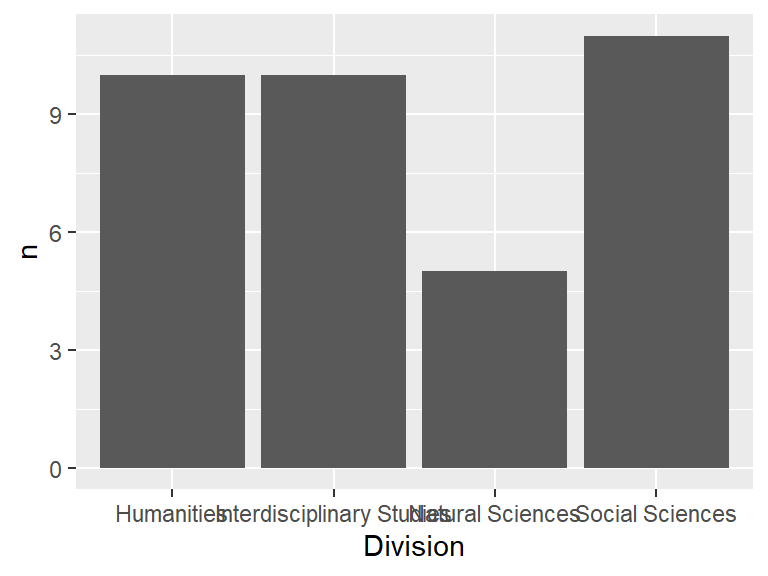The first function, ggplot(), specifies the data table and the variables that will be mapped to the plot window. The aes() function instructs R to map the Division column to the x-axis and the n column to the y-axis. The next function, geom_bar(), specifies the geometry to be used to visualize the data. Its argument, stat = "identity" simply indicates that the values already represent counts.

We can flip the axes by adding coord_flip() to the code chunk.

ggplot(dat2, aes(x = Division, y = n)) + geom_bar(stat = "identity") +
coord_flip()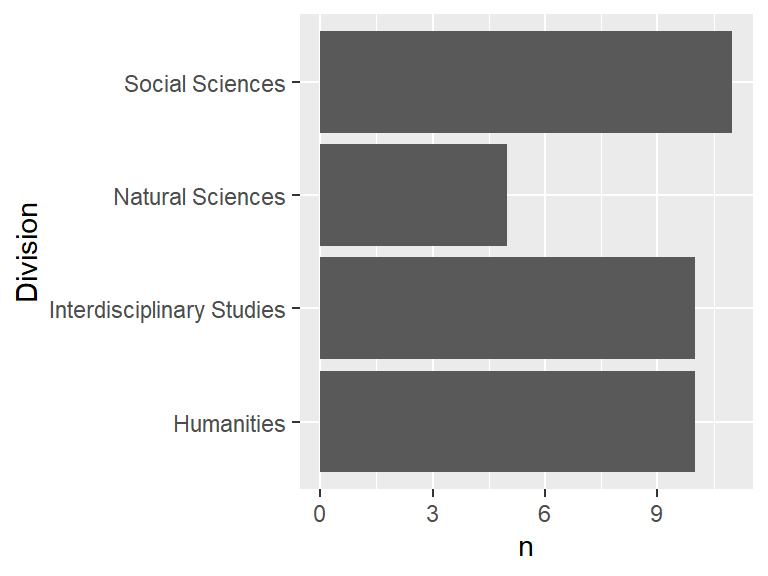We may also opt to reorder the divisions by count values. This requires wrapping the Division variable with the reorder() function which takes, as a second argument, the numeric variable n to be used to reorder the divisions.

ggplot(dat2, aes(x = reorder(Division, n), y = n)) +
geom_bar(stat = "identity") + coord_flip() +
xlab("Division") + ylab("Count")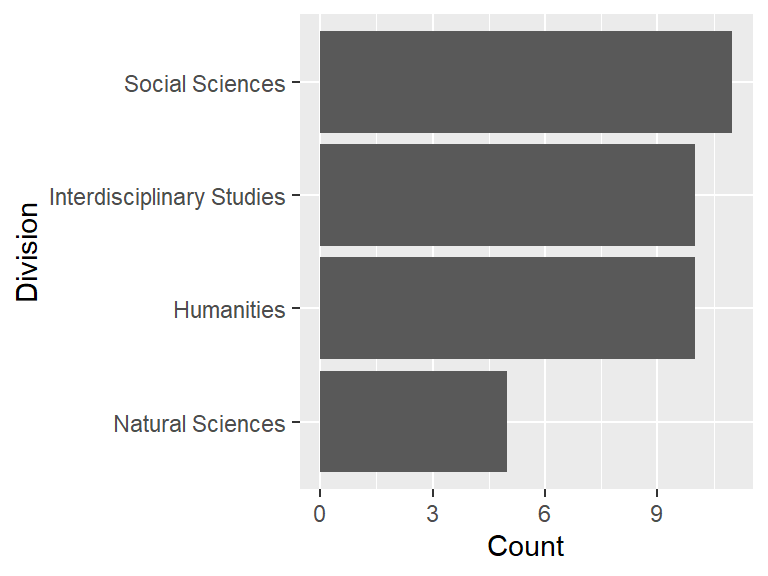### Histogram

In this example, we’ll generate a histogram of registration values from the original courses data file, dat.

ggplot(dat, aes(x = Reg)) + geom_histogram()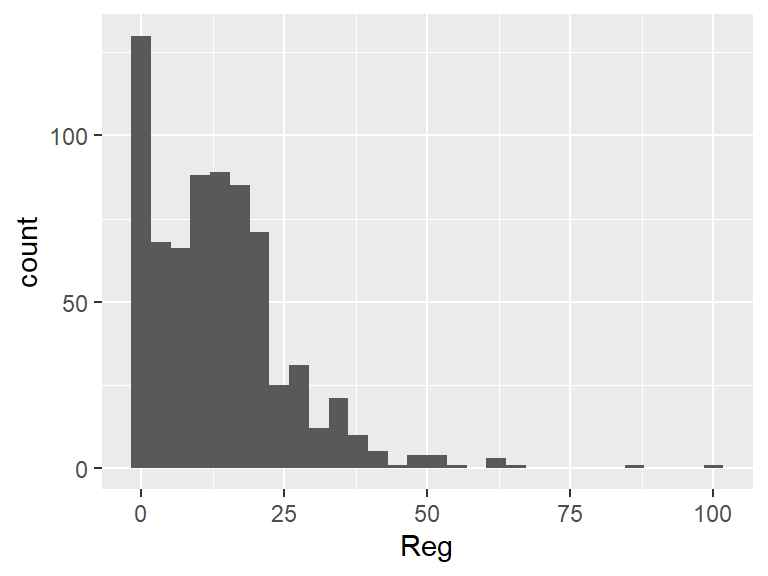### Scatter plot

In this next example, we’ll work off of dat1 and two of its variables (Number and Reg) to generate a scatter plot.

ggplot(dat1, aes(x = Number, y = Reg)) + geom_point()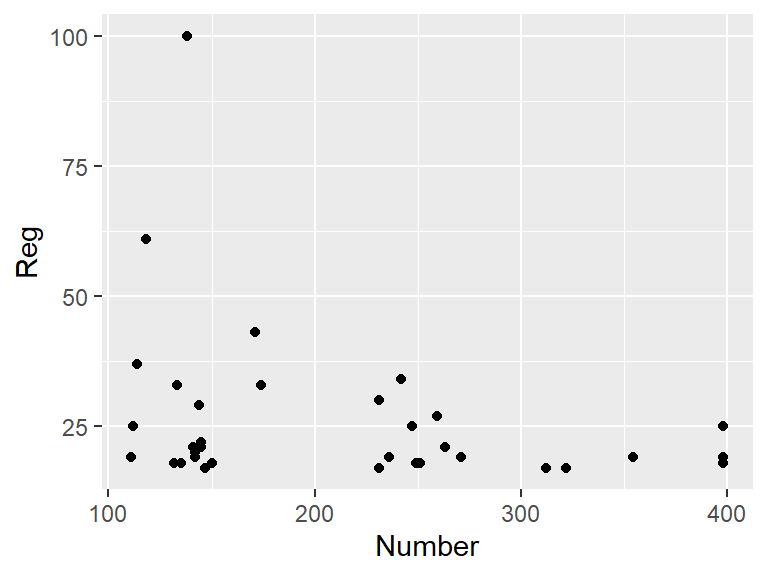### Faceting plots

You can also break the plots down into sub-plots based on a categorical variable. For example, to generate a histogram of class sizes by division, type:

ggplot(dat1, aes(x = Reg)) + geom_histogram() + facet_wrap(~ Division)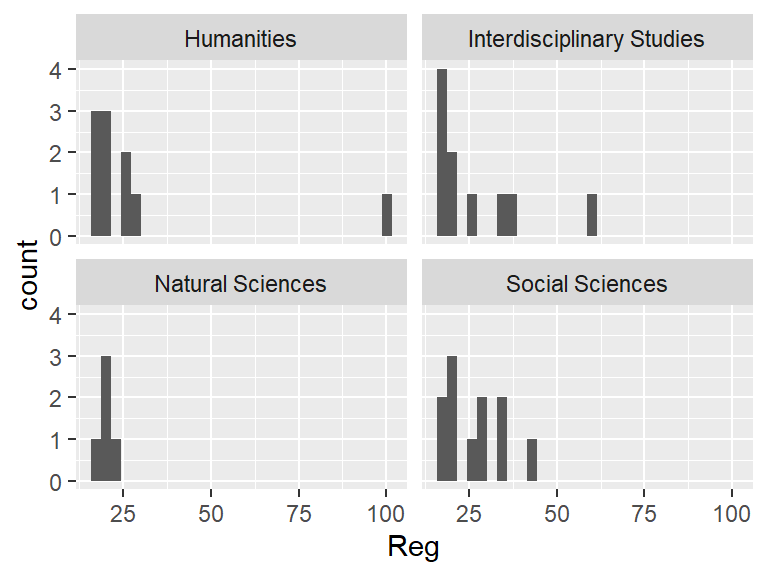The facet_wrap() function instructs ggplot to break the histogram plots into separate plots based on the unique Division values (there are four in our example) associated with each record in dat1.